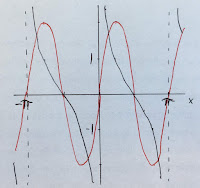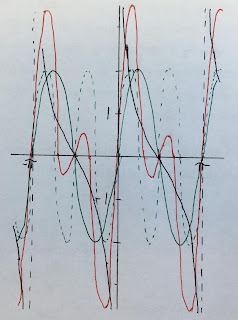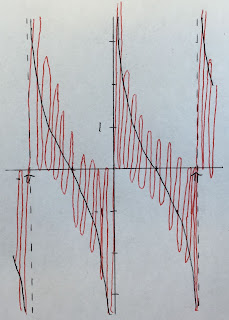## Friday, August 3, 2018

### The Fourier Series of the Cotangent Function

In Section 11.5 of Intermediate Physics for Medicine and Biology, Russ Hobbie and I describe the Fourier series. I’m always looking for illustrative examples of Fourier series to assign as homework (see Problems 10.20 and 10.21), to explain in class, or to include on an exam. Not every function will work; it must be well behaved (in technical jargon, it must obey the Dirichlet conditions). Sometimes, however, I like to examine a case that does not satisfy these conditions, just to see what happens.

Consider the Fourier series for the cotangent function, cot(x) = cos(x)/sin(x).The cotangent function, from Schaum's Outlines: Mathematical Handbook of Formulas and Tables.

The function is periodic with period π, but at zero it asymptotically approaches infinity. Its Fourier series is defined as

where

The cotangent is odd, implying that only sines contribute to the sum and a0 = an = 0. Because the product of two odd functions is even, we can change the lower limit of the integral for bn to zero and multiply the integral by two

To evaluate this integral, I looked in the best integral table in the world (Gradshteyn and Ryzhik) and found

implying that bn = 2, independent of n. The Fourier series of the cotangent is therefore

When I teach Fourier series, I require that students plot the function using just a few terms in the sum, so they can gain intuition about how the function is built from several frequencies. The first plot shows only the first term (red). It's not a good approximation to the cotangent (black), but what can you expect from a single frequency?The cotangent function approximated by a single frequency.
The second plot shows the first term (green, solid), the second term (green dashed), and their sum (red). It’s better, but still has a long ways to go.The cotangent function approximated by two frequencies.
If you add lots of frequencies the fit resembles the third plot (red, first ten terms). The oscillations don’t seem to converge to the function and their amplitude remains large.The cotangent function approximated by ten frequencies.
The Youtube video below shows that the oscillation amplitude never dies down. It is like the Gibbs phenomenon on steroids; instead of narrow spikes near a discontinuity you get large oscillations everywhere.

The bottom line: the Fourier method fails for the cotangent; its Fourier series doesn’t converge. High frequencies contribute as much as low ones, and there are more of them (infinitely more). Nevertheless, we do gain insight by analyzing this case. The method fails in a benign enough way to be instructive.

I hope this analysis of a function that does not have a Fourier series helps you understand better functions that do. Enjoy!